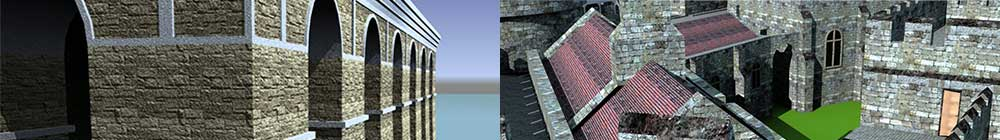SketchUp
Dynamic
Components

# 3. Entering formulas

In the last lesson, we looked at entering values into the Attributes window but what makes a component dynamic is formulas – the ability for some attributes to change depending on others.

Start by creating a cube 10cm on each side (you can use inches if you want – units are not important) and making it into a component. Then open the Component Attributes window, select the cube and add the size attributes (LenX, LenY and LenZ). They should all be showing "10". (If they aren't, just enter 10 into the incorrect attribute and press return).

The first thing we are going to do is fix the height (LenZ) so that it remains fixed at 10 whatever else we do to the cube, such as scaling. This is a common task in dynamic components. All we have to do is type "=10" into the LenZ attribute box (not the quote marks, just equals and ten).

Note: if you simply add the = in front of the existing text, you might see this appear:

This means that SketchUp doesn't recognise what you enter. You have to remove the "cm" so the value you enter is "=10". The "cm" will appear when you press return but that is simply SketchUp showing you the units. You don't enter them yourself.

Now take the scaling tool and try changing the dimensions of the cube. If everything is correct, you should see the height change but snap back to 10cm when you release the mouse. The LenZ attribute is now fixed.

Before we go on, a tip. You can either see the computed values or the formulas in the attributes boxes. To toggle between the two, click the "=ƒx" button at the top right of the Attribute window.

Now we want to link the width (LenY) of the cube to the length (LenX). So delete the contents of the LenY attribute and enter "=LenX/3" and return (again not including the quote marks). What this means is that LenY is set equal to LenX divided by 3. The width of the cube should automatically change to 3.333 cm as soon as you pressed return.

Now try scaling the cube again and you should see the width automatically change to one-third of the length.

If you haven't done so, try scaling just the width using the scaling point below.

You should notice that the cube* remains the same size but simply moves its position. What happened? Well, we have determined that the width is calculated according to the length. We have changed the width but not the length so SketchUp calculates the new width dependant on the length which hasn't changed. The scale tool cannot override the formulas.

You could try switching the length and width around by deleting the LenY formula so that it just shows the value (in grey) and entering "=LenY*3" in the LenX box. Now you see that the scaling tool can change the length but not the width.

When you are entering formulas, you can enter the attribute directly rather than type it by clicking on the attribute name in the window (see below).

What happens if you want to connect the width to the length and the length to the width? If you have the two formulas in both boxes at the same time, you will get the red # coming up to show that SketchUp cannot accept it. It's a circular reference. The LenX value is based on LenY and the LenY is based on LenX. SketchUp, just like a spreadsheet, cannot complete the calculations and will throw a wobbly.

To finish off, try entering various formulas using the position attributes (X, Y and Z). If the formula in position X is "=Y*2", then moving the cube along the X axis will automatically move it to a new Y position. Try entering different formulas in different boxes until you are quite happy with how they work.

* I know it's probably not strictly a cube now but a box, but we won't confuse things by changing the name.